CoCalc Public FilesLab Notebooks / Lab 1: Hydrogen Spectroscopy / Hydrogen Spectroscopy.html
Views : 33
Description: Jupyter html version of Lab Notebooks/Lab 1: Hydrogen Spectroscopy/Hydrogen Spectroscopy.ipynb
Compute Environment: Ubuntu 18.04 (Deprecated)
(File too big to render with math typesetting.)
Hydrogen Spectroscopy

# Pre-Lab Exercises¶

Due at the start of lab on Mon/Tues, Jan 16/17. You may perform your calculations right here in Python, or do the calculations on paper and show them to your TA.

1. Calculate the wavelengths of the Balmer $\alpha$, $\beta$, $\gamma$, and $\delta$ lines in hydrogen and deuterium. Are these lines actually visible?
2. Calculate the isotope shifts $\lambda_H - \lambda_D$ of the Balmer $\alpha,\beta,\gamma$ and $\delta$ lines.
In :
def line(n):
R = 10973731.568639
if(n < 3):
return 1
return (R*(.25 - (1./(n*n))))
me = 9.109383e-28
md = 3.343584e-24
mp = md*.50025
def hydr(n): return (1.+(me/mp))/line(n)
def deut(n): return (1.+(me/md))/line(n)
Ha = hydr(3)
Hb = hydr(4)
Hc = hydr(5)
Hd = hydr(6)
Da = deut(3)
Db = deut(4)
Dc = deut(5)
Dd = deut(6)
def diff(n): return.5*me/mp/line(n)
a = diff(3)
b = diff(4)
c = diff(5)
d = diff(6)
print "Hydrogen lines at ", Ha, Hb, Hc, Hd
print "Deuterium lines at", Da, Db, Dc, Dd
print "lambda separations", a,b,c,d

Hydrogen lines at  6.56469605001e-07 4.86273781482e-07 4.34173019181e-07 4.10293503126e-07
Deuterium lines at 6.56291030028e-07 4.86141503724e-07 4.3405491404e-07 4.10181893767e-07
lambda separations 1.78664305161e-10 1.32343929749e-10 1.1816422299e-10 1.11665190726e-10


# Mon/Tues, January 16/17¶

Place your notes, data, and analysis here...and maybe even a photo or sketch of your experimental setup.

Just to illustrate how to insert an image, I am including the figure from the lab guide here:firstly lens should be chosen such that it matches f of the spectometer, but this was done ahead of time. next grating is fiddled with until peak A is found, at which point the PMT voltage is toned down until multimeter reads below 30mA. labview is used to scan through the grating spacing, through range manually set from observed range around peak. labview recorded output data is in units of twice the wavelength. this was done for all four lines at first for tube of just H, next moving on to deuterium. for gamma line voltage was boosted a lot to compensate for the weakness of the spectral line, seemed to induce significant signal to noise in comparison to alpha and beta lines. likewise with the delta line but even more exacerbated (signal to noise of about 2 or 3:1 at the peak). having some trouble with removing the hydrogen tube, as well as finding the deuterium signal. both deuterium peaks (including H) are much sharper, narrowing band of measurement and increased resolution to get finer grained data. first run looks very good, reducing the slit size and increasing source tube intensity leads to visually similar data with a small artifact in trough between both peaks. trailing line seems to be less sloped than leading with a much slower drop to zero (doesn't appear to approach asymptote as fast or has higher asymptote). switching direction of scan (from high to low wavelength to low to high) retains this (leading switches to trailing) which means it's not a scanning effect. switching back to high to low wavelength to measure other spectral lines starting with beta. beta lines are way too close together, decreasing slit size to make them distinguishable, boosting source tube intensity and PMT voltage to compensate for smaller signal. with this beta line it is even clearer that the trailing end drops off slower, time constant must be significantly higher. changing the orientation of the PMT doubles the measured A? we set PMT orientation to the maximum A which slightly improves the trailing vs leading edge difference. at minimum A orientation, leading edge seems worse, as well as trailing edge, and data is visually much less clean.

## Plans for next time¶

• Bulleted list...
• ...of things to do next

# Wed/Thurs, January 18/19¶

exit slit size of 15 and 21 are where it's possible to see a downward slope from the first (smaller) peak from the deuterium tube. switched out tube to one that has a much larger first peak than the other tube, size of first peak appeared to increase as the day wore on, moreso than as factor of slit size?

# Mon/Tues, January 23/24¶

vastly better deuterium tube data for alpha line, ran a few trials. first beta line trial was a bit worse of a fit (less definition between peaks, weird residual falloff at the ends) but readjustment (closing) of the entry slit size made an impact and now the data is much better, running a few more trials. gamma line peak maxes out at about 5mA even with max pmt voltage and data is a bit rougher than the other lines but signal to noise is still very good. reduced exit slit from 20.5 to 19.5 for second pass. data fit expectation better but error increased. ran 5 more trials on gamma, moving on to delta which peaks out at less than 1mA so data will be very unclean. signal to noise of about 4-5, running 9 more trials to compensate.

# Wed/Thurs, January 25/26¶

In :
# %load Halpha
# slit size 	amperage

%matplotlib inline
import numpy as np
from scipy.optimize import curve_fit
import matplotlib.pyplot as plt
import matplotlib.ticker as mtick
import matplotlib.lines as mlines
import scipy.constants as cs

def line(n):
R = 10973731.568639
if(n < 3):
return 1
return (R*(.25 - (1./(n*n))))
me = 9.109383e-28
md = 3.343584e-24
mp = md*.50025
def hydr(n): return (1.+(me/mp))/line(n)
def deut(n): return (1.+(me/md))/line(n)
Ha = hydr(3)
Hb = hydr(4)
Hc = hydr(5)
Hd = hydr(6)
Da = deut(3)
Db = deut(4)
Dc = deut(5)
Dd = deut(6)
def diff(n): return.5*me/mp/line(n)
a = diff(3)
b = diff(4)
c = diff(5)
d = diff(6)

def gauss(x,a,b,c,d):
return d+(a*np.exp(-((x-b)/c)**2))
def gauss2(x,a1,b1,c1,a2,b2,c2,d):
return d+(a1*np.exp(-((x-b1)/c1)**2))+(a2*np.exp(-((x-b2)/c2)**2))
def lorentz(x,a,b):
return a/(np.pi*((x-b)**2 + a**2))

def graph(filename):
print(filename)
#Make a figure a bit bigger:
fig,ax = plt.subplots(figsize=(10,6.5))
slit = x/1e3
amps = y*1e6
# Turn the frequency data into GHz by dividing by 1e9, and use blue circular markers ('bo'):
ax.plot(slit,amps,'bo',alpha=0.5) # alpha = 0.5 sets the opacity of the markers

#Labels using latex:
ax.set_xlabel(r'Lines per unit length [$1/\mu$m]',size=16)
ax.set_ylabel(r'Output amperage [$\mu$A]',size=16) #The r before the string sets to math mode

#Format x-axis:
ax.xaxis.set_major_formatter(mtick.FormatStrFormatter('%.5f'))

#Show the plot:
mid = len(slit)/2
coefs0, covar0 = curve_fit(gauss, slit, amps, p0=[amps[mid],slit[mid],.003,amps])

# Print the fitting parameter and its statistical uncertainty in pairs.
print("Fit parameters with statistical uncertainties:\n")
print([[coefs0[nn],np.sqrt(covar0[nn,nn])] for nn in range(len(coefs0))])
fit = np.linspace(coefs0-.02,coefs0+.02,200)#+-20kHz around the center frequency
#     fig,ax = plt.subplots(figsize=(14,8))

ax.plot(fit,gauss(fit,*coefs0) ,'-r',label=r'Fit: $P_f = \frac{\mathcal{F}^2}{\mathcal{F}^2 + \delta^2} \sin^2 \left ( \pi \sqrt{\mathcal{F}^2 + \delta^2} t \right)$ ')

plt.show()
return coefs0/2e7

def graph2(filename):
print(filename)
#Make a figure a bit bigger:
fig,ax = plt.subplots(figsize=(10,6.5))
slit = x/1e3
amps = y*1e6
# Turn the frequency data into GHz by dividing by 1e9, and use blue circular markers ('bo'):
ax.plot(slit,amps,'bo',alpha=0.5) # alpha = 0.5 sets the opacity of the markers

#Labels using latex:
ax.set_xlabel(r'Lines per unit length [$1/\mu$m]',size=16)
ax.set_ylabel(r'Output amperage [$\mu$A]',size=16) #The r before the string sets to math mode

#Format x-axis:
ax.xaxis.set_major_formatter(mtick.FormatStrFormatter('%.5f'))

#Show the plot:

mid = len(slit)/2
coefs0, covar0 = curve_fit(gauss2, slit, amps, p0=[amps[mid-1],slit[mid-1],.003,amps[mid+2],slit[mid+3],.002,amps])

# Print the fitting parameter and its statistical uncertainty in pairs.
print("Fit parameters with statistical uncertainties:\n")
print([[coefs0[nn],np.sqrt(covar0[nn,nn])] for nn in range(len(coefs0))])
fit = np.linspace(coefs0-.02,coefs0+.02,200)#+-20kHz around the center frequency
#     fig,ax = plt.subplots(figsize=(14,8))

ax.plot(fit,gauss2(fit,*coefs0) ,'-r',label=r'Fit: $P_f = \frac{\mathcal{F}^2}{\mathcal{F}^2 + \delta^2} \sin^2 \left ( \pi \sqrt{\mathcal{F}^2 + \delta^2} t \right)$ ')

plt.show()
return coefs0/2e7, coefs0/2e7, (coefs0-coefs0)/2e7

print graph('Halpha'), '<measured, predicted>', Ha
print graph('Halpha_2'), '<measured, predicted>', Ha
print graph('Hbeta'), '<measured, predicted>', Hb
print graph('Hgamma'), '<measured, predicted>', Hc
print graph('Hdelta'), '<measured, predicted>', Hd
def HD(n,line, H, D, l):
for i in range(1,n+1):
print graph2('HD_'+line+'_pass'+str(i))
print 'Hydrogen line, Deuterium line, '+line+' separation (^measured, vpredicted)'
print H, D, l
HD(4,'alpha',Ha, Da, a)
HD(5,'beta',Hb, Db, b)
HD(7,'gamma',Hc, Dc, c)
HD(9,'delta',Hd, Dd, d)

Halpha
Fit parameters with statistical uncertainties:

[[25.05723362155118, 0.48820217011496103], [13.096888161195299, 5.1881410733670112e-05], [0.0033375722671496974, 7.8409122161785842e-05], [0.070280954110776034, 0.14679378125294154]]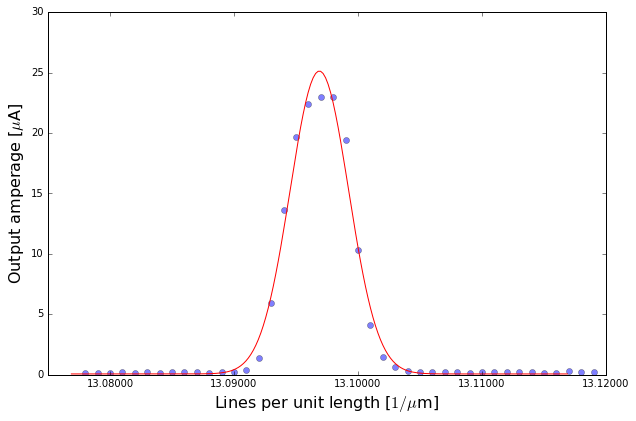6.5484440806e-07 <measured, predicted> 6.56469605001e-07
Halpha_2
Fit parameters with statistical uncertainties:

[[22.74433200522888, 0.30416092611025253], [13.097340858907822, 3.5653280221198688e-05], [0.0033445586469746124, 5.4011333511465205e-05], [0.07029080575192119, 0.093108106579335367]]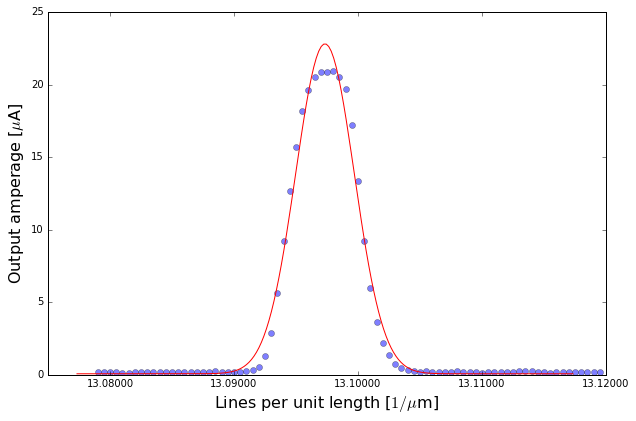6.54867042945e-07 <measured, predicted> 6.56469605001e-07
Hbeta
Fit parameters with statistical uncertainties:

[[28.332954684668465, 0.40357121250063516], [9.6800704349672753, 4.6039042970595741e-05], [0.0040883576042987958, 7.1321599246497778e-05], [0.43219912621461193, 0.14266394312451447]]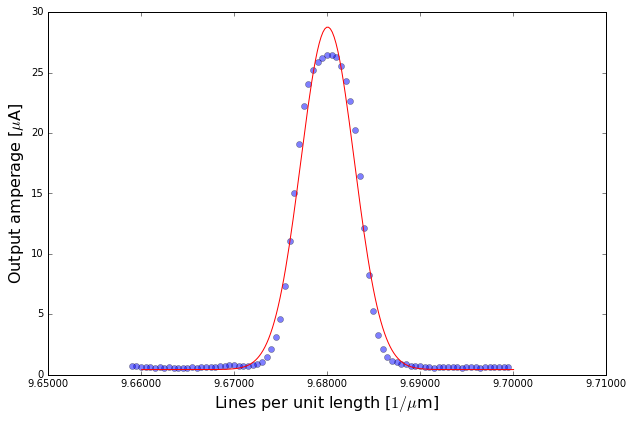4.84003521748e-07 <measured, predicted> 4.86273781482e-07
Hgamma
Fit parameters with statistical uncertainties:

[[13.241095932481807, 0.16940202190485912], [8.6376202857376061, 4.1044371340179677e-05], [0.0040564700175685061, 6.3518150024339493e-05], [3.3380882000934116, 0.059533761141295077]]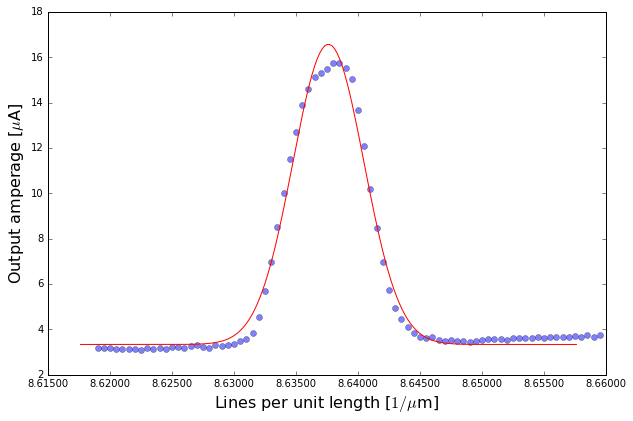4.31881014287e-07 <measured, predicted> 4.34173019181e-07
Hdelta
Fit parameters with statistical uncertainties:

[[0.76258763819752684, 0.017889807545350366], [8.1587640360357891, 7.8114674742498438e-05], [0.0042187341564042984, 0.00012153465482124651], [0.60087907530612306, 0.0064758933617974574]]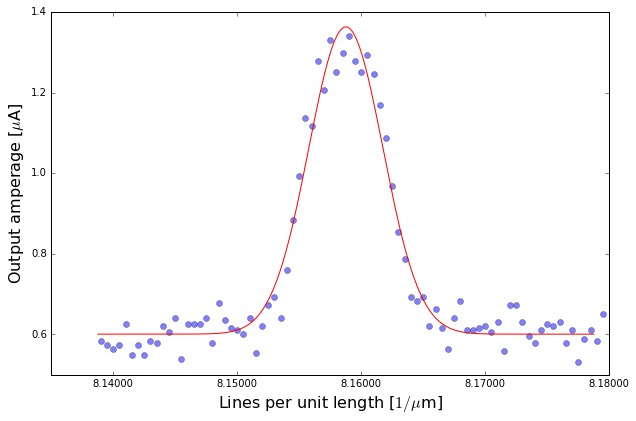4.07938201802e-07 <measured, predicted> 4.10293503126e-07
HD_alpha_pass1
Fit parameters with statistical uncertainties:

[[2.1018002237309936, 0.020488983049331808], [13.094807535201602, 1.5445745415100127e-05], [0.0014357717148925481, 2.1506374294930482e-05], [1.36101485282177, 0.018500632976640043], [13.098472607422588, 2.673687993848203e-05], [0.0018344705900189778, 4.0500989349343358e-05], [0.15730474072058534, 0.0043219906409639706]]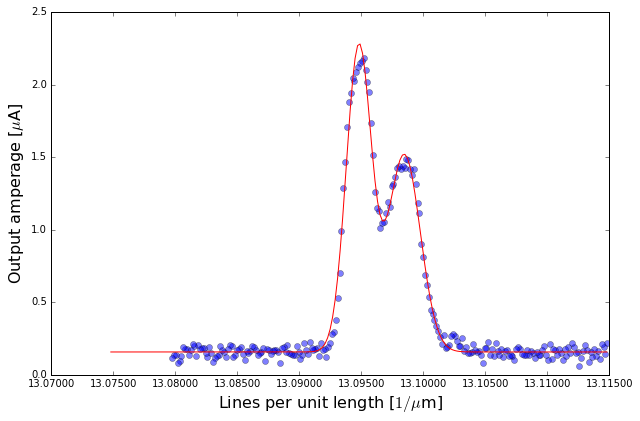(6.5474037676008013e-07, 6.5492363037112942e-07, 1.832536110493166e-10)
Hydrogen line, Deuterium line, alpha separation (^measured, vpredicted)
6.56469605001e-07 6.56291030028e-07 1.78664305161e-10
HD_alpha_pass2
Fit parameters with statistical uncertainties:

[[2.0175446724268706, 0.019882953190015117], [13.094651243074956, 1.5443035547335011e-05], [0.0014085938807199747, 2.1246694968298163e-05], [1.3164651418122124, 0.017576470882691549], [13.098288351823676, 2.71116845280396e-05], [0.001870366922717414, 4.1068536439299327e-05], [0.15389692168588018, 0.0041379378275099342]]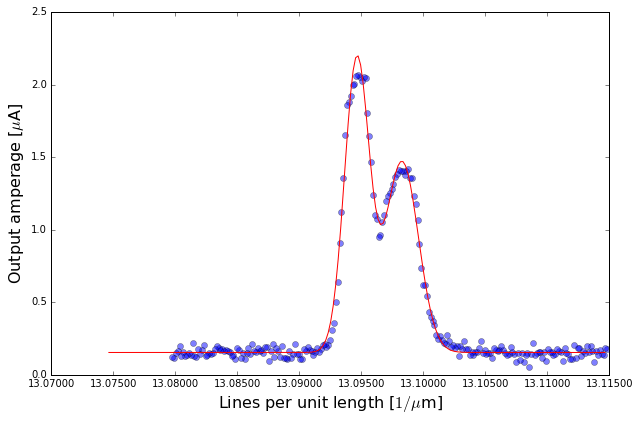(6.5473256215374783e-07, 6.5491441759118385e-07, 1.8185543743598488e-10)
Hydrogen line, Deuterium line, alpha separation (^measured, vpredicted)
6.56469605001e-07 6.56291030028e-07 1.78664305161e-10
HD_alpha_pass3
Fit parameters with statistical uncertainties:

[[1.99766725808515, 0.019617757609302181], [13.09450053046937, 1.5751895406623971e-05], [0.0014362074288120006, 2.1744692908257969e-05], [1.2805813074256174, 0.017553424022958702], [13.098164382586686, 2.7791371851686672e-05], [0.0018615743785494478, 4.1970558527922737e-05], [0.15250049495591453, 0.0041324152479716532]]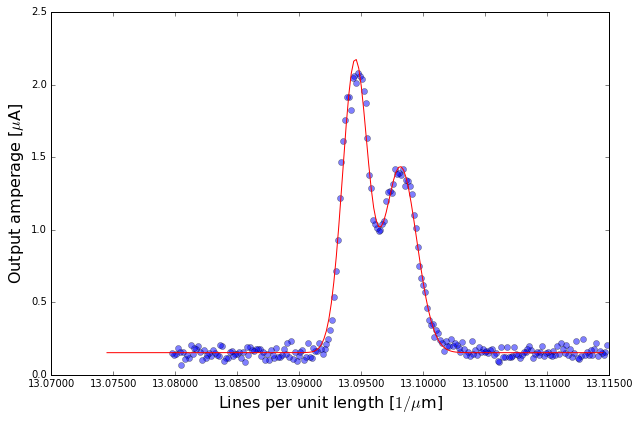(6.5472502652346848e-07, 6.5490821912933434e-07, 1.8319260586583397e-10)
Hydrogen line, Deuterium line, alpha separation (^measured, vpredicted)
6.56469605001e-07 6.56291030028e-07 1.78664305161e-10
HD_alpha_pass4
Fit parameters with statistical uncertainties:

[[24.927951442919237, 0.14409958622253979], [13.094258278178362, 4.9419855269074328e-06], [0.0010523543348403663, 7.2100974431975865e-06], [12.039331770088246, 0.13597815857218995], [13.097845847610609, 1.0856296362683015e-05], [0.001187676629297968, 1.5922143824671805e-05], [0.19472835420921358, 0.025373287962182606]]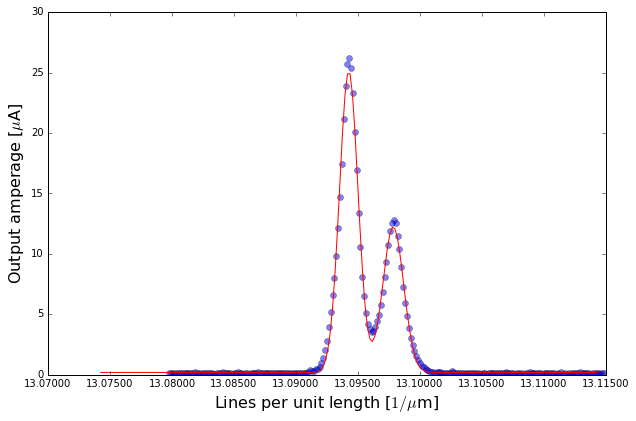(6.5471291390891812e-07, 6.5489229238053046e-07, 1.7937847161233478e-10)
Hydrogen line, Deuterium line, alpha separation (^measured, vpredicted)
6.56469605001e-07 6.56291030028e-07 1.78664305161e-10
HD_beta_pass1
Fit parameters with statistical uncertainties:

[[11.920881285260521, 0.32168774167976028], [9.6817422934423725, 3.739144328137702e-05], [0.0030420141289644086, 6.1334308796968877e-05], [11.313281696190925, 0.40101336172717927], [9.6811306925494467, 1.7580651375911208e-05], [0.00075640432639099476, 3.2468458194229447e-05], [0.055005901194178496, 0.049122927764651853]]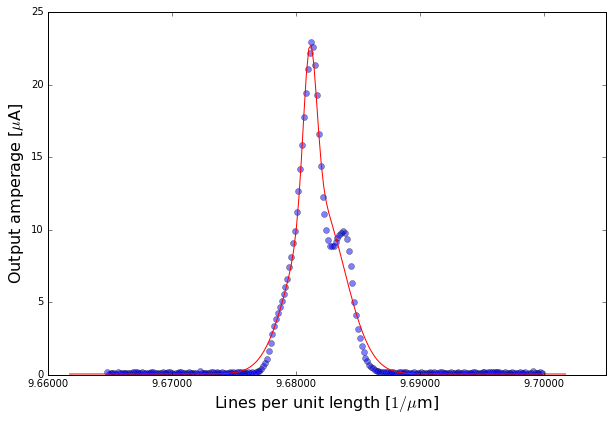(4.8408711467211863e-07, 4.840565346274723e-07, -3.0580044646288942e-11)
Hydrogen line, Deuterium line, beta separation (^measured, vpredicted)
4.86273781482e-07 4.86141503724e-07 1.32343929749e-10
HD_beta_pass2
Fit parameters with statistical uncertainties:

[[23.571970845519015, 0.11448289986379402], [9.6802186521476621, 3.3179853305281125e-06], [0.00081829129873928501, 4.8951409724194134e-06], [12.101317383216008, 0.10419443671400735], [9.6828464244291208, 7.1146714243884243e-06], [0.0010040553596529819, 1.0688969818677465e-05], [0.17066654656736674, 0.017225864919276749]]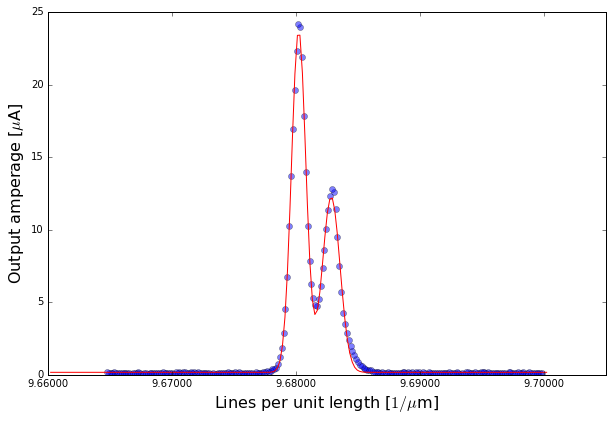(4.8401093260738308e-07, 4.8414232122145607e-07, 1.3138861407293589e-10)
Hydrogen line, Deuterium line, beta separation (^measured, vpredicted)
4.86273781482e-07 4.86141503724e-07 1.32343929749e-10
HD_beta_pass3
Fit parameters with statistical uncertainties:

[[12.19624020839529, 0.10670701771734185], [9.6826317130178623, 7.1859230695555501e-06], [0.00099837784696593613, 1.0785980492196607e-05], [23.416526437234499, 0.11696810334367123], [9.6800055861261889, 3.4080315753460118e-06], [0.00081817366662919439, 5.0274353369751355e-06], [0.17494485396445314, 0.017594409764447067]]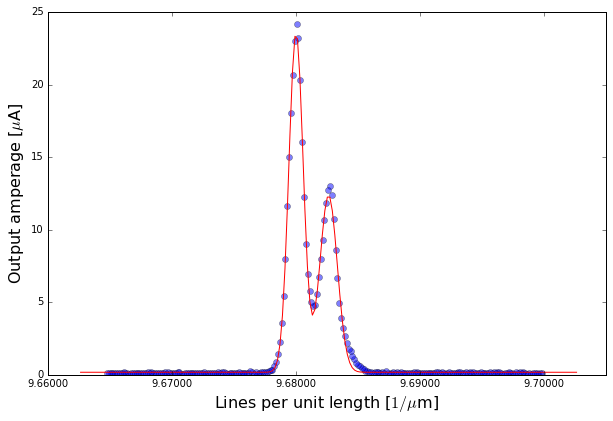(4.8413158565089311e-07, 4.8400027930630945e-07, -1.3130634458367042e-10)
Hydrogen line, Deuterium line, beta separation (^measured, vpredicted)
4.86273781482e-07 4.86141503724e-07 1.32343929749e-10
HD_beta_pass4
Fit parameters with statistical uncertainties:

[[13.05543269341066, 0.11032896959098606], [9.6825234785347369, 6.8934285675966279e-06], [0.00099436526301275164, 1.0320643151839626e-05], [25.978407529529907, 0.12174216023892616], [9.6798989137093177, 3.1337143758428976e-06], [0.0008042057515506983, 4.6123096769077201e-06], [0.17340504504438797, 0.018158438615726129]]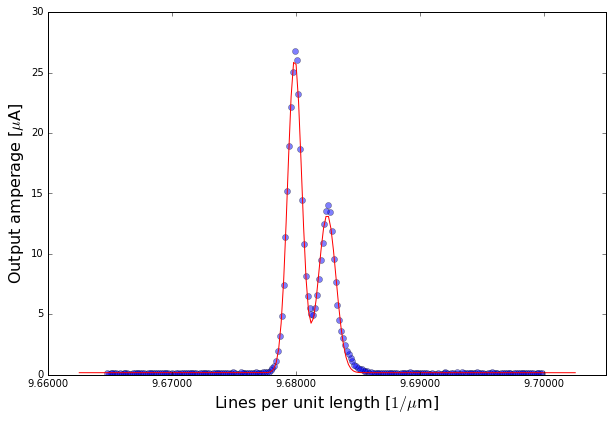(4.8412617392673686e-07, 4.8399494568546589e-07, -1.3122824127096421e-10)
Hydrogen line, Deuterium line, beta separation (^measured, vpredicted)
4.86273781482e-07 4.86141503724e-07 1.32343929749e-10
HD_beta_pass5
Fit parameters with statistical uncertainties:

[[25.394310879244934, 0.12068674521482299], [9.6797044082350236, 3.1855820376822656e-06], [0.00080617674482967062, 4.6897422005861044e-06], [12.955357184129904, 0.10955472924644369], [9.6823294381069402, 6.8917504026569199e-06], [0.00099309401756209189, 1.0318135918374693e-05], [0.17211264143226301, 0.018021650837122823]]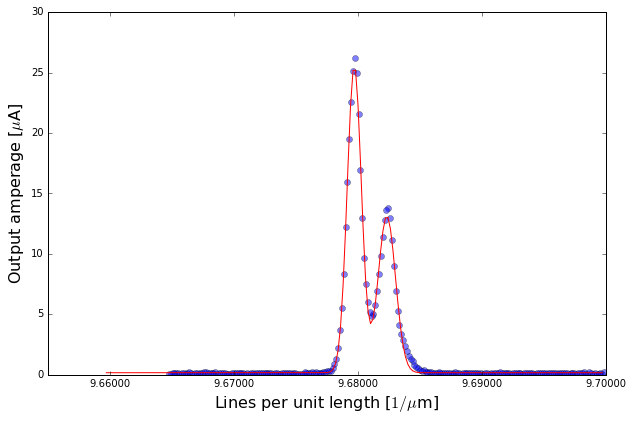(4.8398522041175119e-07, 4.8411647190534702e-07, 1.3125149359582976e-10)
Hydrogen line, Deuterium line, beta separation (^measured, vpredicted)
4.86273781482e-07 4.86141503724e-07 1.32343929749e-10
HD_gamma_pass1
Fit parameters with statistical uncertainties:

[[3.3651288948107583, 0.026900266471205799], [8.6395009055908041, 8.1831757442038638e-06], [0.0010472442718741709, 1.2529080863044769e-05], [5.3970059698145896, 0.028624361278923895], [8.6371913859549778, 4.7640081369409049e-06], [-0.00089399424689847774, 6.9665425069865672e-06], [0.14384497222542686, 0.0044887159740868814]]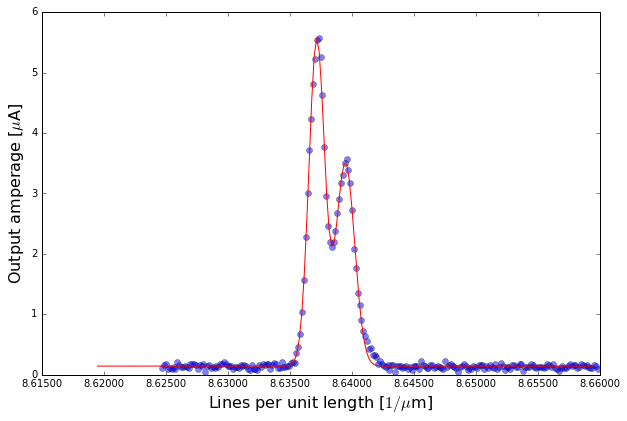(4.319750452795402e-07, 4.3185956929774889e-07, -1.1547598179131313e-10)
Hydrogen line, Deuterium line, gamma separation (^measured, vpredicted)
4.34173019181e-07 4.3405491404e-07 1.1816422299e-10
HD_gamma_pass2
Fit parameters with statistical uncertainties:

[[2.7918692293761276, 0.026254017006363262], [8.63933935806668, 9.4042616238593328e-06], [-0.001033670841399541, 1.4394509687641498e-05], [4.4788718809711501, 0.027715161166730534], [8.637020269388783, 5.5230201622387146e-06], [0.00090021778821754433, 8.1286027375439769e-06], [0.13829017206835706, 0.0043561939643922878]]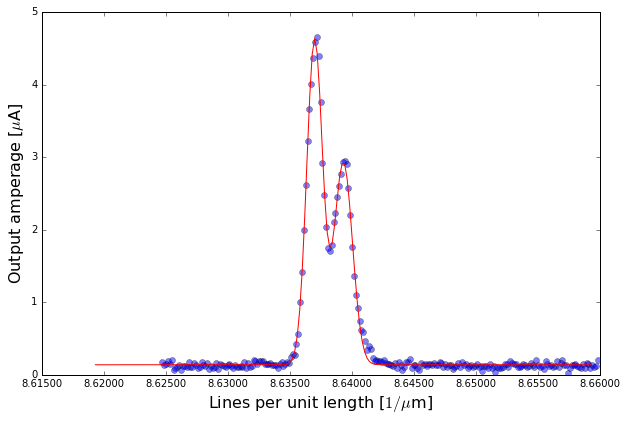(4.3196696790333399e-07, 4.3185101346943917e-07, -1.1595443389484928e-10)
Hydrogen line, Deuterium line, gamma separation (^measured, vpredicted)
4.34173019181e-07 4.3405491404e-07 1.1816422299e-10
HD_gamma_pass3
Fit parameters with statistical uncertainties:

[[4.5076088636645357, 0.02801603674603247], [8.6368802553166049, 5.3843555986511919e-06], [0.00089335954251495292, 7.978291911873863e-06], [2.8110217049234363, 0.026643130636863178], [8.6392002511977815, 9.11989219811637e-06], [0.0010148952034595069, 1.3984133895627106e-05], [0.14053242818930192, 0.0043793848222090995]]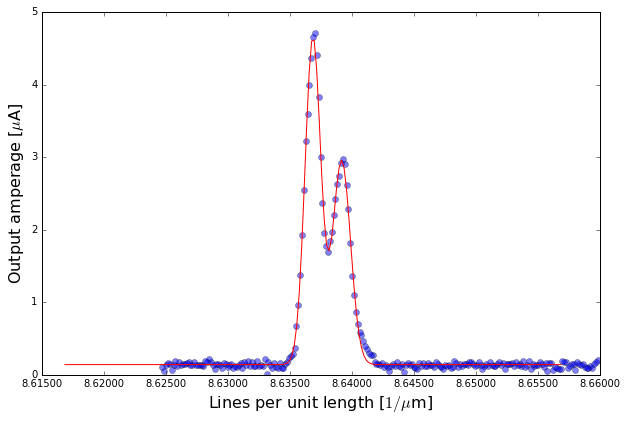(4.3184401276583026e-07, 4.319600125598891e-07, 1.1599979405882977e-10)
Hydrogen line, Deuterium line, gamma separation (^measured, vpredicted)
4.34173019181e-07 4.3405491404e-07 1.1816422299e-10
HD_gamma_pass4
Fit parameters with statistical uncertainties:

[[2.7997000095819344, 0.028022679222983519], [8.6390471873386829, 1.0022173218959045e-05], [0.0010399191968814025, 1.5374296994195783e-05], [4.4826818915766413, 0.029760878758913499], [8.6367264796725287, 5.8592398797711956e-06], [0.00089231684411356668, 8.6109579739406599e-06], [0.13739025796307613, 0.0046599642192035884]]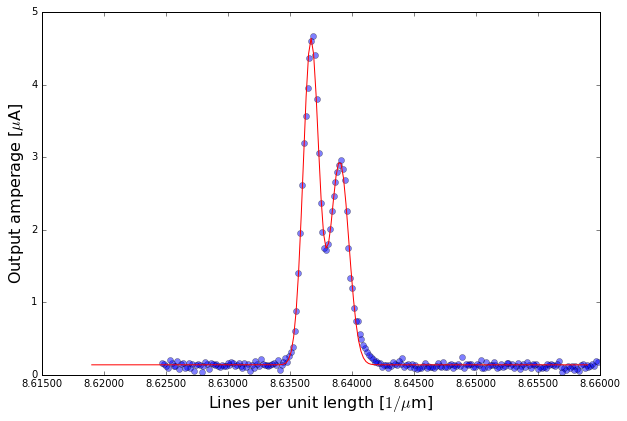(4.3195235936693412e-07, 4.3183632398362642e-07, -1.1603538330771102e-10)
Hydrogen line, Deuterium line, gamma separation (^measured, vpredicted)
4.34173019181e-07 4.3405491404e-07 1.1816422299e-10
HD_gamma_pass5
Fit parameters with statistical uncertainties:

[[4.4813236892657624, 0.027791487427049542], [8.6365781848622358, 5.3233997933054453e-06], [-0.00089194371014884887, 7.9088001769028475e-06], [2.8419406320589018, 0.02650738007736721], [8.6388961350349973, 8.8366994573136238e-06], [0.0010054627321393625, 1.3549142783378955e-05], [0.14145842838373335, 0.0043370358424805336]]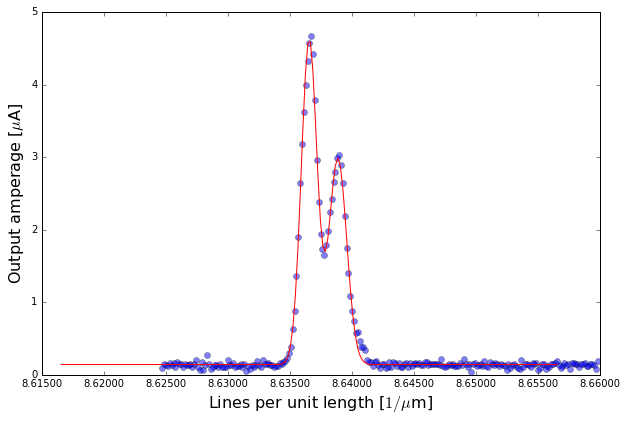(4.3182890924311178e-07, 4.3194480675174985e-07, 1.1589750863807424e-10)
Hydrogen line, Deuterium line, gamma separation (^measured, vpredicted)
4.34173019181e-07 4.3405491404e-07 1.1816422299e-10
HD_gamma_pass6
Fit parameters with statistical uncertainties:

[[2.5395692967759453, 0.026076031990706929], [8.6387605802958447, 1.04805623695249e-05], [0.0010499288001620997, 1.6059607645929433e-05], [4.0726880244010424, 0.027734400629106513], [8.6364363512151314, 6.1059076780533016e-06], [0.00089732912667796932, 8.9441163839427742e-06], [0.13538500236009132, 0.0043584065074933395]]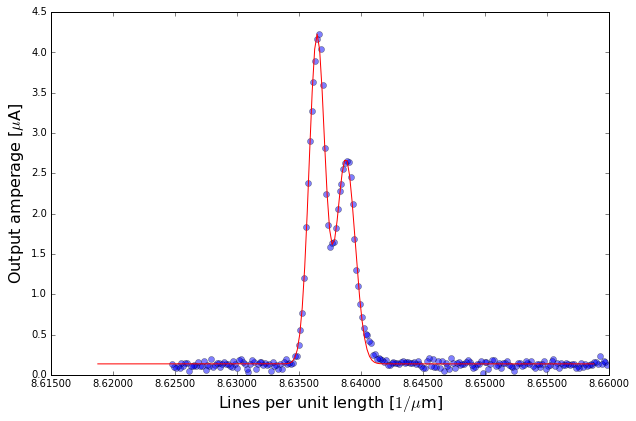(4.3193802901479225e-07, 4.3182181756075655e-07, -1.1621145403566401e-10)
Hydrogen line, Deuterium line, gamma separation (^measured, vpredicted)
4.34173019181e-07 4.3405491404e-07 1.1816422299e-10
HD_gamma_pass7
Fit parameters with statistical uncertainties:

[[3.138783372022306, inf], [8.6370743803476717, inf], [-0.002257574287749592, inf], [-0.0098289137184894709, inf], [8.6474769108443077, inf], [-3.8409056390496105e-06, inf], [0.11706956274758346, inf]]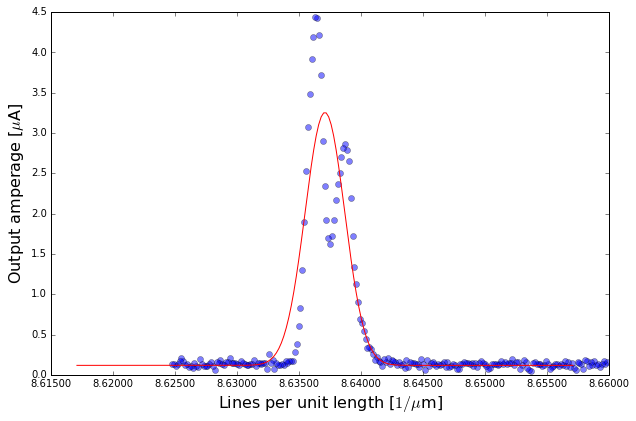(4.3185371901738359e-07, 4.3237384554221541e-07, 5.2012652483179879e-10)
Hydrogen line, Deuterium line, gamma separation (^measured, vpredicted)
4.34173019181e-07 4.3405491404e-07 1.1816422299e-10
HD_delta_pass1
Fit parameters with statistical uncertainties:

[[-0.29115353398789201, 0.047481186512605446], [8.157896382803365, 6.599458449515729e-05], [0.00092361207292795337, 0.00015063519156593183], [0.5365942806898979, 0.018497841446317768], [8.1600652267083476, 0.00014906081041595826], [0.0026059058797497725, 0.00015016628032473705], [0.12701696624814635, 0.0040979909332308504]]